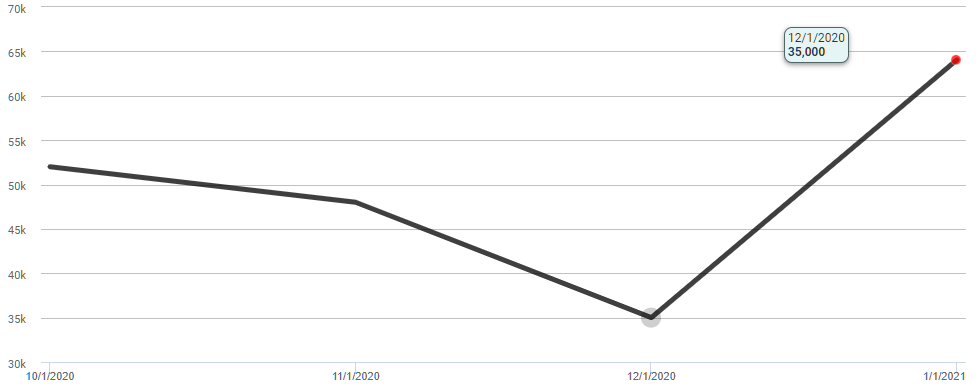•Since we don't know what type of X-Axis data (number, category, datetime), the easiest way is to make sure your data is sorted so that it matches the X-Axis order, add a sequence column, an aggregate column set to Max, and a calculated column which uses a simple iff statement.  Use that calculated column as an @Chart token value for the data point color.

`<Report ID="LogiForum.serieslastpoint">  <Body>    <ChartCanvas ID="cc1">      <Series Type="Line" Color="Black" LineThickness="4" ChartXDataColumn="Name" ChartXDataColumnType="DateTime" ChartYDataColumn="Value">        <DataLayer Type="Static">          <StaticDataRow Name="10/1/2020" Value="52000" />          <StaticDataRow Name="11/1/2020" Value="48000" />          <StaticDataRow Name="12/1/2020" Value="35000" />          <StaticDataRow Name="1/1/2021" Value="64052" />          <SequenceColumn ID="seqid" />          <SortFilter SortColumn="seqid" DataType="Number" />          <AggregateColumn AggregateColumn="seqid" AggregateFunction="Max" ID="MaxName" DataType="Date" />          <CalculatedColumn Formula="iif(&quot;@Data.seqid~&quot; == &quot;@Data.MaxName~&quot;, &quot;#FF0000&quot;, &quot;Transparent&quot;)" ID="Color" />        </DataLayer>        <MarkerPoints MarkerPointFillColor="@Chart.Color~" MarkerPointRadius="5" />      </Series>      <ChartXAxis>        <AxisLabelStyle Format="Short Date" />      </ChartXAxis>    </ChartCanvas>  </Body>  <ideTestParams /></Report>`•Thank you for this but I want to show the Label so what the value is at the last point - is this possible?

•My apologies for misunderstanding your question.  The two-series solution you have would be the easiest method.  It does not appear that the Data Label element accepts a tokenized color based on the row of data for the point.

The only other way to achieve this would be to utilize javascript with the beforeCreateChart or afterCreateChart events.

https://devnet.logianalytics.com/hc/en-us/articles/4419715103511-Events-beforeCreateChart-and-afterCreateChart